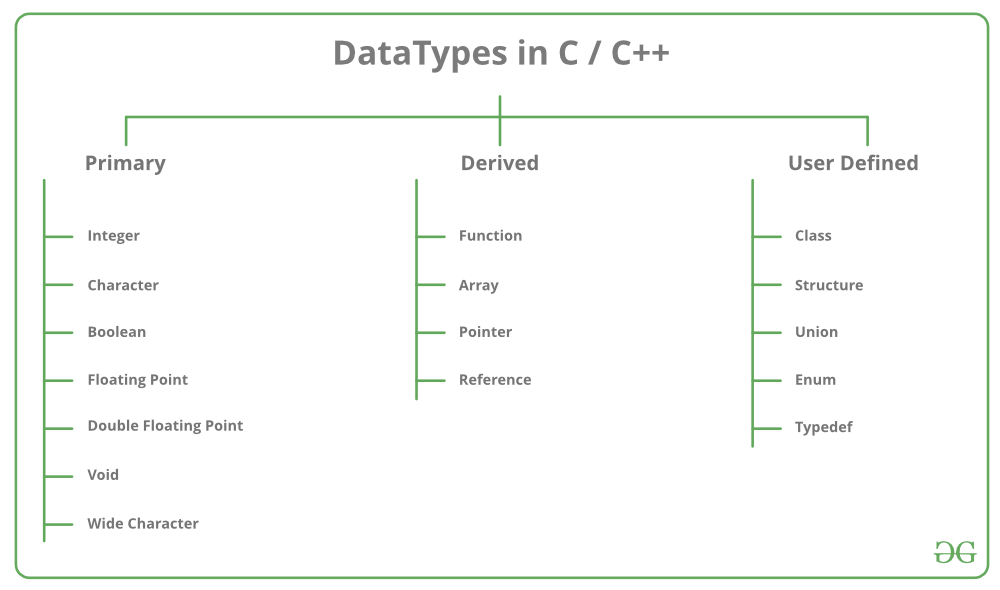# Derived Data Types in C++

• Difficulty Level : Easy
• Last Updated : 26 Feb, 2020

Data types are means to identify the type of data and associated operations of handling it. There are three types of data types:

1. Pre-defined DataTypes
2. Derived Data Types
3. User-defined DataTypes### Derived Data Types

The data-types that are derived from the primitive or built-in datatypes are referred to as Derived Data Types. These can be of four types namely:

Let’s briefly understand each of the following derived datatypes:

1. Function: A function is a block of code or program-segment that is defined to perform a specific well-defined task. A function is generally defined to save the user from writing the same lines of code again and again for the same input. All the lines of code are put together inside a single function and this can be called anywhere required. main() is a default function that is defined in every program of C++.

Syntax:

`FunctionType FunctionName(parameters)`

Example:

 `// C++ program to demonstrate``// Function Derived Type`` ` `#include ``using` `namespace` `std;`` ` `// max here is a function derived type``int` `max(``int` `x, ``int` `y)``{``    ``if` `(x > y)``        ``return` `x;``    ``else``        ``return` `y;``}`` ` `// main is the default function derived type``int` `main()``{``    ``int` `a = 10, b = 20;`` ` `    ``// Calling above function to``    ``// find max of 'a' and 'b'``    ``int` `m = max(a, b);`` ` `    ``cout << ``"m is "` `<< m;``    ``return` `0;``}`

Output:

```m is 20
```
2. Array: An array is a collection of items stored at continuous memory locations. The idea of array is to represent many instances in one variable.Syntax:

`DataType ArrayName[size_of_array];`

Example:

 `// C++ program to demonstrate``// Array Derived Type`` ` `#include ``using` `namespace` `std;``int` `main()``{`` ` `    ``// Array Derived Type``    ``int` `arr;``    ``arr = 5;``    ``arr = -10;`` ` `    ``// this is same as arr = 2``    ``arr[3 / 2] = 2;`` ` `    ``arr = arr;`` ` `    ``cout<

Output:

```5 2 -10 5
```
3. Pointers: Pointers are symbolic representation of addresses. They enable programs to simulate call-by-reference as well as to create and manipulate dynamic data structures. It’s general declaration in C/C++ has the format:

Syntax:

`datatype *var_name;`

Example:

```int *ptr;

which holds int data```

Example:

 `// C++ program to illustrate``// Pointers Derived Type`` ` `#include ``using` `namespace` `std;`` ` `void` `geeks()``{``    ``int` `var = 20;`` ` `    ``// Pointers Derived Type``    ``// declare pointer variable``    ``int``* ptr;`` ` `    ``// note that data type of ptr``    ``// and var must be same``    ``ptr = &var;`` ` `    ``// assign the address of a variable``    ``// to a pointer``    ``cout << ``"Value at ptr = "``         ``<< ptr << ``"\n"``;``    ``cout << ``"Value at var = "``         ``<< var << ``"\n"``;``    ``cout << ``"Value at *ptr = "``         ``<< *ptr << ``"\n"``;``}`` ` `// Driver program``int` `main()``{``    ``geeks();``}`

Output:

```Value at ptr = 0x7ffc10d7fd5c
Value at var = 20
Value at *ptr = 20
```
4. Reference: When a variable is declared as reference, it becomes an alternative name for an existing variable. A variable can be declared as reference by putting ‘&’ in the declaration.

Example:

 `// C++ program to illustrate``// Reference Derived Type`` ` `#include ``using` `namespace` `std;`` ` `int` `main()``{``    ``int` `x = 10;`` ` `    ``// Reference Derived Type``    ``// ref is a reference to x.``    ``int``& ref = x;`` ` `    ``// Value of x is now changed to 20``    ``ref = 20;``    ``cout << ``"x = "` `<< x << endl;`` ` `    ``// Value of x is now changed to 30``    ``x = 30;``    ``cout << ``"ref = "` `<< ref << endl;`` ` `    ``return` `0;``}`

Output:

```x = 20
ref = 30
```

My Personal Notes arrow_drop_up